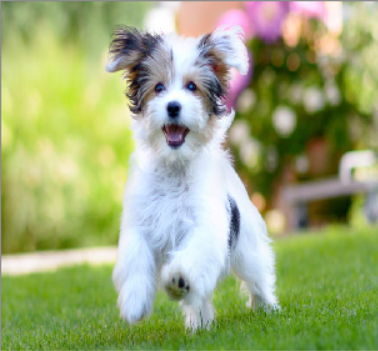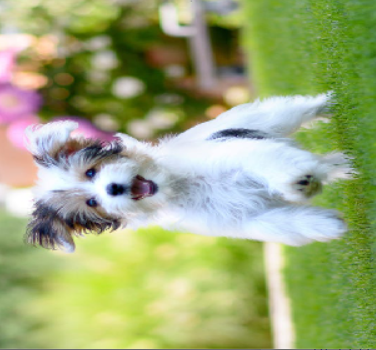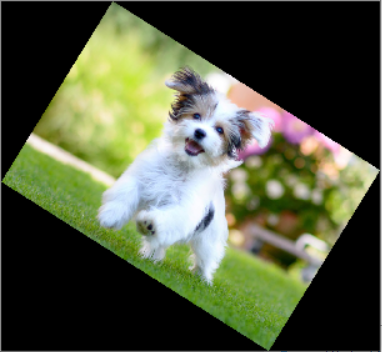# How to Rotate Image by Angle in Python with OpenCV and imutils Libraries## Introduction

In this tutorial, we will show you how to rotate the image by any angle with the help of OpenCV and imutils libraries. We are going to cover the following functions in the post with examples –

1. OpenCV function cv2.rotate() to rotate the image by 90 degrees and 180 degrees
2. imutils function rotate_bound() and rotate() to rotate the image by any angle

## Rotate Image using OpenCV : cv2.rotate()

### Syntax

cv2.rotate(src, rotateCode)

• src – Input Image on which rotation has to be applied
• rotateCode – Its a code to specify how to rotate the image

## Examples of cv2.rotate() in Python OpenCV

First of all, let us import the OpenCV library as shown below-

In :

`import cv2`

### Read Sample Image and Display

Next, we will read a sample image for our example and display it

In :

```window_name='Dog Image'
cv2.namedWindow(window_name, cv2.WINDOW_NORMAL)
cv2.imshow(window_name,img)
cv2.waitKey(0)
cv2.destroyAllWindows()```

Output### Example – 1: Rotate the Image 90 degree clockwise with cv2.rotate()

To rotate the image 90 degree clockwise, use the rotateCode = cv2.ROTATE_90_CLOCKWISE as shown below.

In :

```rotate_image = cv2.rotate(img, cv2.ROTATE_90_CLOCKWISE)

window_name='OpenCV Rotate Image 90 degree Clockwise'
cv2.namedWindow(window_name, cv2.WINDOW_NORMAL)
cv2.imshow(window_name,rotate_image)
cv2.waitKey(0)
cv2.destroyAllWindows()```

Output### Example – 2: Rotate the Image 180 degree with cv2.rotate()

To rotate the image 90 degree clockwise, use the rotateCode = cv2.ROTATE_180 as shown in the below example. There is no concept of rotating the image clockwise or counterclockwise here because the rotation of the image by 180 brings the transformed image to the same state in both cases.

In :

```rotate_image = cv2.rotate(img, cv2.ROTATE_180)

window_name='OpenCV Rotate Image 180 degree'
cv2.namedWindow(window_name, cv2.WINDOW_NORMAL)
cv2.imshow(window_name,rotate_image)
cv2.waitKey(0)
cv2.destroyAllWindows()```

Output### Example – 3: Rotate the Image 90 degree counterclockwise or 270 degrees clockwise

To rotate the Image 90 degree counterclockwise or 270 degrees clockwise (which is the same thing) we use rotateCode= ROTATE_90_COUNTERCLOCKWISE as shown in the below example.

In :

```rotate_image = cv2.rotate(img, cv2.ROTATE_90_COUNTERCLOCKWISE)

window_name='OpenCV Rotate Image 90 degree anticlockwise'
cv2.namedWindow(window_name, cv2.WINDOW_NORMAL)
cv2.imshow(window_name,rotate_image)
cv2.waitKey(0)
cv2.destroyAllWindows()```

Output## Rotate Image by Angle in Python using imutils Library

The out of the box OpenCV function cv2.rotate() only lets us rotate the image in multiple of 90 degrees. However, there is no direct function to rotate the image by any degree in OpenCV, it is a multistep process. This is where imutils library can be useful.

imutils library consists of many convenient wrapper functions on top of OpenCV. It contains two useful functions imutils.rotate() and imutils.rotate_bound() to rotate the image by any given angle.

The difference between the two functions is that imutils.rotate() may end up cropping the image while rotating it whereas imutils.rotate_bound() does not crop the image and preserves the entire image within the bound.

### Syntax for imutils.rotate()

imutils.rotate(image, angle)

• image – Input Image on which rotation has to be applied
• angle – The angle at which the image has to be rotated

### Syntax for imutils.rotate_bound()

imutils.rotate_bound(image, angle)

• image – Input Image on which rotation has to be applied
• angle – The angle at which the image has to be rotated

### Example to Rotate Image by Angle using imutils.rotate()

In the below example we have used imutils.rotate() to rotate the image by 32 degrees. It can be noticed the image is cropped after rotation is applied.

In :

```import imutils

rotate_image = imutils.rotate(img, 32)
window_name='Rotate Image by Angle in Python'
cv2.namedWindow(window_name, cv2.WINDOW_NORMAL)
cv2.imshow(window_name,rotate_image)
cv2.waitKey(0)
cv2.destroyAllWindows()```

Output### Example to Rotate Image by Angle using imutils.rotate_bound()

In the below example we have used imutils.rotate_bound() to rotate the image by 32 degrees. This time it can be seen that the image is not cropped and is preserved in entirety within the bounds.

In :

```import imutils

rotate_image = imutils.rotate_bound(img, 32)
window_name='Rotate Image by Angle in Python'
cv2.namedWindow(window_name, cv2.WINDOW_NORMAL)
cv2.imshow(window_name,rotate_image)
cv2.waitKey(0)
cv2.destroyAllWindows()```

Output